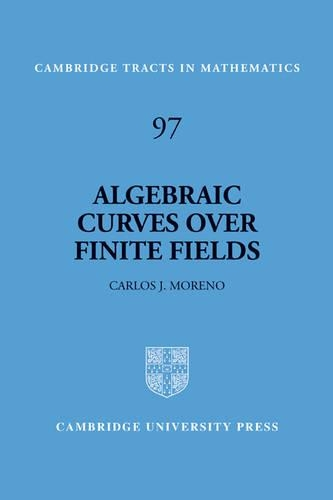•# Algebraic Curves over Finite Fields - Cambridge Tracts in Mathematics (Paperback)

(author)
£46.99
Paperback 260 Pages / Published: 14/10/1993
• Not available

This product is currently unavailable.

In this Tract Professor Moreno develops the theory of algebraic curves over finite fields, their zeta and L-functions, and, for the first time, the theory of algebraic geometric Goppa codes on algebraic curves. Amongst the applications considered are: the problem of counting the number of solutions of equations over finite fields; Bombieri's proof of the Reimann hypothesis for function fields, with consequences for the estimation of exponential sums in one variable; Goppa's theory of error-correcting codes constructed from linear systems on algebraic curves. There is also a new proof of the Tsfasman-Vladut-Zink theorem. The prerequisites needed to follow this book are few, and it can be used for graduate courses for mathematics students. Electrical engineers who need to understand the modern developments in the theory of error-correcting codes will also benefit from studying this work.

Publisher: Cambridge University Press
ISBN: 9780521459013
Number of pages: 260
Weight: 390 g
Dimensions: 229 x 152 x 15 mm

MEDIA REVIEWS
' ... a careful and comprehensive guide to some of the most fascinating of plasma processes, a treatment that is both thorough and up-to-date.' The Observatory
"...well written and has many interesting exercises. It could be used as a textbook for a nice course addressed to students with some background in algebra or number theory." Jose Felipe Voloch, Mathematical Reviews
"This book is well written and has many interesting exercises. It could be used as a textbook for a nice course addressed to students with some background in algebra or number theory." Jose Felipe Voloch, Mathematical Reviews